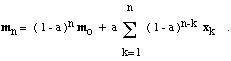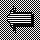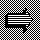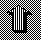# Sequential k-Means Clustering

Another way to modify the k-means procedure is to update the means one example at a time, rather than all at once. This is particularly attractive when we acquire the examples over a period of time, and we want to start clustering before we have seen all of the examples. Here is a modification of the k-means procedure that operates sequentially:
• Make initial guesses for the means m1, m2, ..., mk
• Set the counts n1, n2, ..., nk to zero
• Until interrupted
• Acquire the next example, x
• If mi is closest to x
• Increment ni
• Replace mi by mi + (1/ni)*( x - mi)
• end_if
• end_until
It is a good exercise to show that the resulting mi is the average of all of the examples x that were closest to mi when they were acquired.

This also suggests another alternative in which we replace the counts by constants. In particular, suppose that a is a constant between 0 and 1, and consider the following variation:
• Make initial guesses for the means m1, m2, ..., mk
• Until interrupted
• Acquire the next example, x
• If mi is closest to x , replace mi by mi + a*( x - mi )
• end_until
Electrical engineers familiar with DSP will say that we have replaced an averaging operation by a low-pass-filtering operation. The result might be called the "forgetful" sequential k-means procedure. It is not hard to show that mi is a weighted average of the examples that were closest to mi, where the weight decreases exponentially with the "age" to the example. To be more precise, if mo is the initial value of mi and if xj is the jth of n examples that were used to form mi, then it is not hard to show thatThus, the initial value mo is eventually forgotten, and recent examples receive more weight than ancient examples. This variation of k-means is particularly simple to implement, and it is attractive when the nature of the problem changes over time and the cluster centers "drift." It also leads naturally to the idea of self-organizing feature maps.Back to Fuzzy k-MeansOn to SOFMUp to Clustering